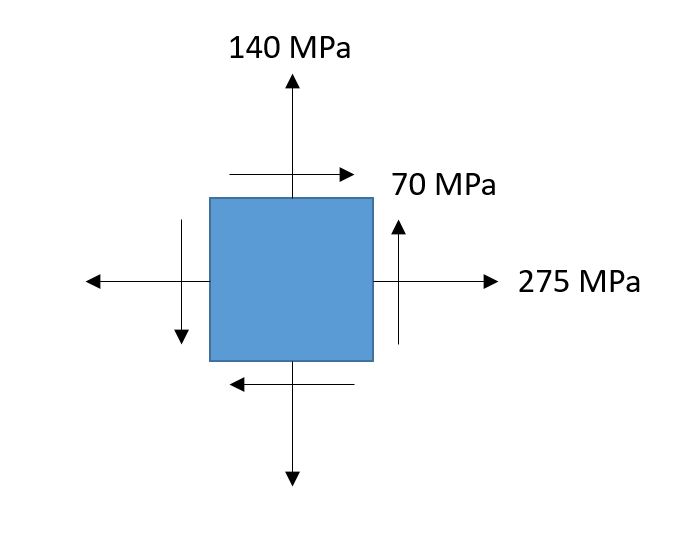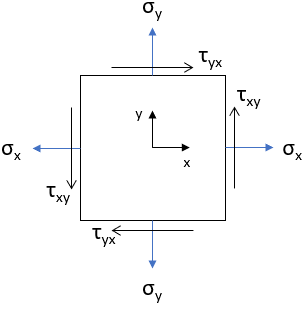## In-plane Shear Stress

In the element below, what is the max in-plane shear stress?Hint
Mohr's Circle:
$$R=\sqrt{(\frac{\sigma _{x}-\sigma_{y}}{2})^{2}+\tau_{xy}^{2}}$$$Hint 2 $$\sigma_x$$ , $$\sigma_y$$ , and $$\tau_{xy}$$ are defined as:From a constructed Mohr's Circle, the max in-plane shear stress occurs when $$\tau_{max}=R$$ $$R=\sqrt{(\frac{\sigma _{x}-\sigma_{y}}{2})^{2}+\tau_{xy}^{2}}$$$Thus, the max in-plane shear stress is:
$$R=\sqrt{(\frac{275-140}{2})^{2}+70^{2}}$$$$$R=\sqrt{4,556.25+4,900}=97.24\:MPa$$$
97.24 MPa